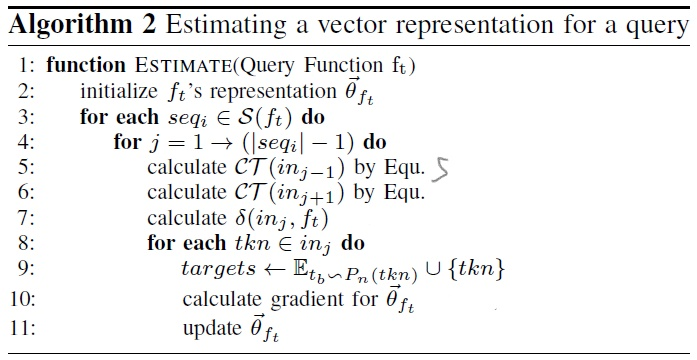# S&P-2019-Asm2Vec

Contents

## 论文概要

• 参照NLP中的PV-DM模型，该模型基于tokens学习文档的表示 Doc2Vec

• 区别：文档是顺序排列的（sequentially laid out），汇编代码可被视作有特定语法的图（a graph and has a specific syntax）。

## PV-DM 模型

• 中间一个词作为目标（target），而两边的词作为上下文（context），完成多分类任务。
• 通过段落和词的ID，将它们分别映射到一个对应的向量上。
• 把这些向量加和平均后（经激活函数sigmod），使用多类别分类器（如softmax）预测目标词。
• 分类错误被反向传播，用于更新词和段落向量。

$\sum_{p}^{T}\sum_{s}^{p}\sum_{t=k}^{\vert{s}\vert-k}logP(w_t\vert{p},w_{t-k},...,w_{t+k}) \tag{1}$

## Asm2Vec

Assembly code carries richer syntax than plaintext. It contains operations, operands, and control flow that are structurally different than plaintext.

These differences require a different model architecture design that cannot be addressed by PV-DM. Next, we present a representation learning model that integrates the syntax of assembly code.

### CFG序列化

1. 选择性内联被调用函数

原因：函数内联，即编译器将指定的函数体插入并取代每一处调用该函数的地方（上下文），从而节省了每次调用函数带来的额外时间开支。该优化会很大程度的改变CFG，给汇编克隆搜索带来了很大的挑战。

本文选择满足以下条件的被调用函数进行內联：

• 非库函数。因为模型能较好地获取library call token的词法语义（lexical semantic）。
• 只內联图上的一阶被调用函数。递归內联会过度扩展caller。
• 沿用 BinGo 的 decoupling metric（式2）和阈值（0.01）。
• caller大于十行指令时，以式3的 $$\delta<0.6$$ 过滤太长的被调用函数。caller（$$f_s$$）小于十行指令时保留內联，是为了容纳包装函数（wrapper function）。
$\alpha(f_c)=outdegree(f_c)/(outdegree(f_c)+indegree(f_c)) \tag{2}$ $\delta(f_s,f_c)=length(f_c)/length(f_s) \tag{3}$
2. 边采样

从 callee-expanded CFG 上随机采样边，直到每条边都被覆盖。连接每条边前后的汇编代码形成序列。这种做法可以在CFG中的基本块被拆分或合并的情况下，仍能产生相似的序列。

3. 随机游走

该方法获得的序列比2中长很多，同时提升了Dominator的基本块的优先级。（Dominator是控制流分析中的概念：若基本块B向下执行必须经过基本块A，则称A dominates B）

### Asm2Vec模型

$\sum_{f_s}^{RP}\sum_{seq_i}^{S(f_s)}\sum_{in_j}^{seq_i}\sum_{t_c}^{\tau(in_j)}logP(t_c\vert{p,in_{j-1},in_{j+1}}) \tag{4}$

• 每个标识（token）被映射为 $$d$$ 维的向量 $$v_t^{\rightarrow}$$ ，以及 $$2\times{d}$$ 维的映射 $$v_{t'}^{\rightarrow}$$。前者用于生成上下文指令的向量，后者用于（目标指令）标识的预测。

• 每条指令 $$in$$ 表示为操作码 $$d$$ 维向量与 $$d$$ 维操作数向量均值的连接，即 $$2\times{d}$$ 维的 $$C\tau{(in)}$$ ，如式5所示。

$C\tau{(in)}=v_{P_{(in)}}^{\rightarrow}\vert\vert\frac{1}{\vert{A(in)}\vert}\sum_t^{A(in)}v_{t_b}^{\rightarrow} \tag{5}$
• 每个函数 $$f_s$$ 被映射为与指令同维度的 $$2\times{d}$$ 维向量，记作 $$\theta_{f_s}^{\rightarrow}$$ 。
• $$\theta_{f_s}^{\rightarrow}$$ 和 $$v_t^{\rightarrow}$$ 的元素初始化为接近0的较小随机值， $$v_{t'}^{\rightarrow}$$ 初始化为全0向量。

#### Training

$$\theta_{f_s}^{\rightarrow}$$ 与目标指令前后两条指令的向量平均后得 $$\delta(in_j,f_s)$$ ，则式4中的概率项可表示为 $$P(t_c\vert\delta(in_j,f_s))$$ 。

$P(t_c\vert\delta(in_j,f_s))=P(v_{t'_c}^{\rightarrow}\vert\delta(in_j,f_s))=\frac{f(v_{t'_c}^{\rightarrow},\delta(in_j,f_s))}{\sum_d^Df(v_{t'_d}^{\rightarrow},\delta(in_j,f_s))} \tag{6}$

#### 训练加速

$\sum_d^Df(v_{t'_d}^{\rightarrow},\delta(in_j,f_s))\approx{\sum_{i=1}^kE_{d_t\sim{P_n(t_c)}}([t_d\neq{t_c}]f(v_{t'_d}^{\rightarrow},\delta(in_j,f_s)))} \tag{7}$

#### Estimating## My Review

$\sum_{f_s}^{RP}\sum_{seq_i}^{S(f_s)}\sum_{in_j}^{seq_i}logP(in_j\vert{p,in_{j-1},in_{j+1}}) \tag{8}$

Updated on